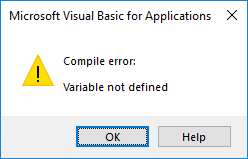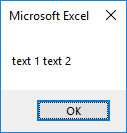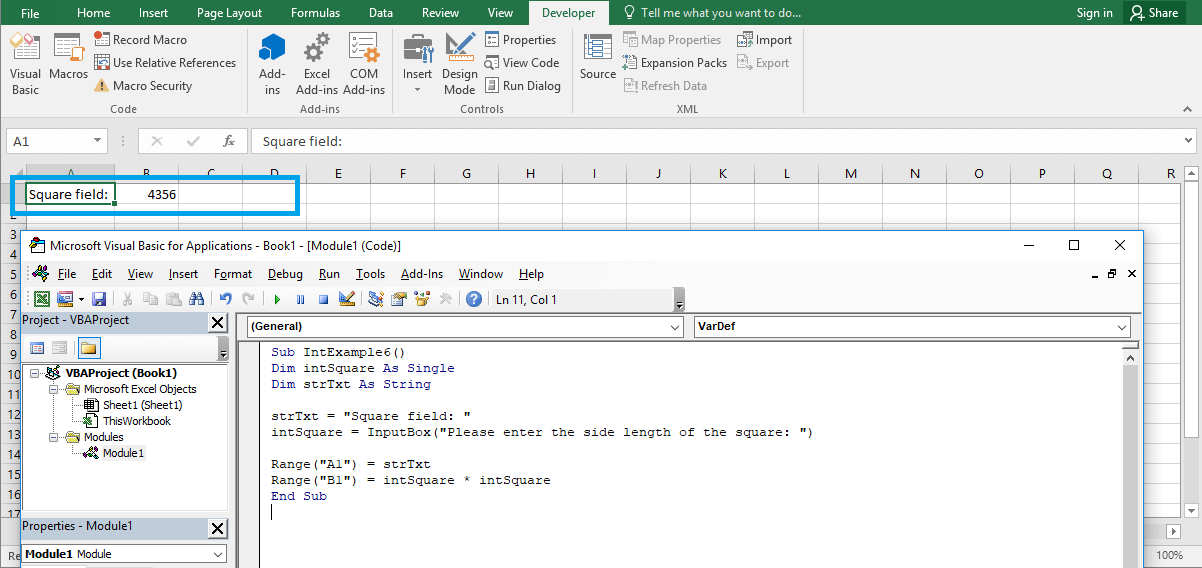# Excel VBA Variables

## Introduction to VBA variables

In VBA, as in other programming languages, we work with variables. The VBA language does not require declaring variables. You can, for example enter variable name that will be interpreted as a variable, and then you can execute any instructions on it. I put an example listed below.

```'OfficeInside.Org
Sub VarDef1()
MsgBox (MyVar * 5)
End Sub```Excel VBA course – VBA variables

The result of the example operation is 0 and means that the variable has received the numeric type at the beginning. We know this because the default value for numeric types is 0. For what then should we define VBA variables? The correct definition of variables is one of the principles of good programming. It avoids future conflicts in your code.

You also avoid cases when variables in you code operation can get an unwanted results. It is also a good rule to add a prefix specifying the data type to the variable name, ie. intNum, StrText, datStart, etc.

If you want the VisualBasic editor to inform you every time you use an undeclared variable, you should write the short code Option Explicit before the name of your program. The VB editor will return an error message when you run the code with undeclared variables.

```Option Explicit
'OfficeInside.Org
Sub VarDef2()
varNotDeclared = 3
End Sub```Excel VBA course – Declare variables VBA

## How to declare VBA variables

You can declare variables in the VBA code by adding the phrase  Dim before the variable name and adding the correct data type at the end. This is called explicit declaration of variables . The phrase Dim informs you, that the variable has been declared at the level of the Sub() procedure.

In the later, code of the program, need to avoid conflicts, ie. if you try to assign text value to the numeric data type, etc. All available data types in Excel VBA can be found in this chapter: VBA data types.

```'OfficeInside.Org
Sub VarDef3()
Dim intVar As Integer
Dim datVar as Date
Dim strVar as String
MsgBox (intVar * 5)
End Sub```Excel VBA course – How to declare VBA variables

## Assigning values ​​to variables in VBA Excel

Ok, you already know what declaring variables is and what data types are. Let’s try to make a simple example by defining variables, their data types and then assigning the right values ​​to them. You can assign values ​​to a declared variable by entering the variable name, equal sign and the value that you want to assign. The example is listed below:

```'OfficeInside.Org
Sub VarDef4()
Dim intVar As Integer
Dim datVar As Date
Dim strVar As String

intVar = 100
datVar = "2016/01/01"
strVar = "simple text message"

MsgBox (intVar)
MsgBox (datVar)
MsgBox (strVar)

End Sub```

In the example listed above, you assigned a date to the previously declared varDat variable. You can see that the date entry mask in the VB code looks like “YYYY/MM/DD”. As you can see, yue assign values ​​for numeric variables without quotes. Variables for text and dates require quotes.Excel VBA course – String. date and numeric variables in VBA

## Actions on variables in VBA Excel

At the moment when your VBA variables are already defined and have assigned values, you can perform any actions on them using the VBA code. Let’s make a simple example using variables.

We want to connect the text string with another text string. With the numeric value, we want to do simple arithmetic operation. In the case of a date value, let’s do a simple function that returns the month from date. Finally, let’s display the example using the VBA MsgBox window.

```'OfficeInside.Org
Sub VarDef5()
Dim intVar As Integer
Dim datVar As Date
Dim strVar As String

intVar = 100
datVar = "2016/01/01"
strVar = "text 1 "
strVar2 = "text 2 "

MsgBox (intVar * 10)
MsgBox (Month(datVar))
MsgBox (strVar + strVar2)
End Sub```Excel VBA course – Operations on variables in VBA

## Levels of variable declarations

Ok, you have declared variables at the level of the procedure by adding the phrase Dim before the variable name. You can also use the Static, Public, Private declarations to declare space for values. The description of all declarations is described in this table:Excel VBA course – VBA Variables. Private, public, dim declaration types

## An example of using a numeric variable

Let’s write a short program calculating the square field based on the length of its side. The program should call the VBA InputBox window, in which you will enter the length of the side of the square. The program in cell A1 of the sheet will insert text value value “Square field:” and in B2 cell, the result of this operation.

```'OfficeInside.Org
Sub IntExample6()
Dim intSquare As Single
Dim strTxt As String

strTxt = "Square field: "
intSquare = InputBox("Please enter the side length of the square: ")

Range("A1") = strTxt
Range("B1") = intSquare * intSquare
End Sub```Excel VBA course – VBA Variables. Calculating square field

File with all examples is attached here: excel_vba_tutorial_variables_in_vba.xlsm

## Tasks (You can enter the solution in a comment)

1. Modify the example from task with calculating square field, displaying the result using a MsgBox message.
2. Create and test a variable declared at the public level. See if it also works in the code of other modules and procedures.

More examples of Excel VBA basics can be found in the Excel VBA Tutorial section and  Excel VBA Examples section. If you have any questions about this chapter, you can post them in comments.